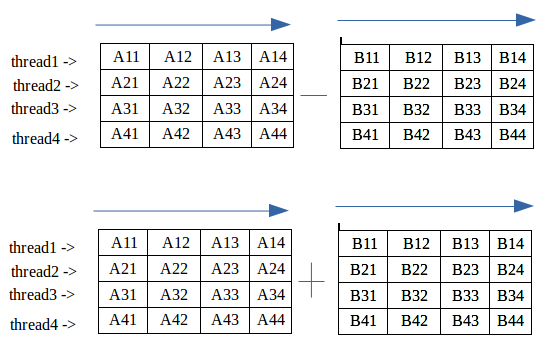# Addition and Subtraction of Matrix using pthreads

• Difficulty Level : Hard
• Last Updated : 18 Apr, 2019

Addition or Subtraction of matrices takes O(n^2) time without threads but using threads we don’t reduce the time complexity of the program we divide the task into core like if we have 4 core then divide the matrix into 4 part and each core take one part of the matrix and compute the operations and finally when each task is complete then all 4 thread join the main program and show final output.

Note –This method only works when we have more then one CPU core if we have only one core the Don’t create the thread because we divide the task and perform on only one CPU so it is waste of time to dividing a task and then compute.Examples:

```Input :

Matrix A:
3 7 3 6
9 2 0 3
0 2 1 7
2 2 7 9

Matrix B:
6 5 5 2
1 7 9 6
6 6 8 9
0 3 5 2

Output :

Sum of Matrix A and B:
9   12   8   8
10   9   9   9
6   8   9   16
2   5   12   11

Subtraction of Matrix A and B:
-3   2  -2   4
8  -5  -9  -3
-6  -4  -7  -2
2  -1   2   7
```

## Recommended: Please try your approach on {IDE} first, before moving on to the solution.

Code – It is advised to execute the program in Linux based system.

 `#include ``#include ``#include `` ` `// Value depend on System core``#define CORE 4`` ` `// Maximum matrix size``#define MAX 4`` ` `// Maximum threads is equal to total core of system``pthread_t ``thread``[CORE * 2];``int` `mat_A[MAX][MAX], mat_B[MAX][MAX], sum[MAX][MAX], sub[MAX][MAX];`` ` `// Addition of a Matrix``void``* addition(``void``* arg)``{`` ` `    ``int` `i, j;``    ``int` `core = (``int``)arg;`` ` `    ``// Each thread computes 1/4th of matrix addition``    ``for` `(i = core * MAX / 4; i < (core + 1) * MAX / 4; i++) {`` ` `        ``for` `(j = 0; j < MAX; j++) {`` ` `            ``// Compute Sum Row wise``            ``sum[i][j] = mat_A[i][j] + mat_B[i][j];``        ``}`` ` `    ``}`` ` `}`` ` ` ` `// Subtraction of a Matrix``void``* subtraction(``void``* arg)``{`` ` `    ``int` `i, j;``    ``int` `core = (``int``)arg;`` ` `    ``// Each thread computes 1/4th of matrix subtraction``    ``for` `(i = core * MAX / 4; i < (core + 1) * MAX / 4; i++) {`` ` `        ``for` `(j = 0; j < MAX; j++) {`` ` `            ``// Compute Subtract row wise``            ``sub[i][j] = mat_A[i][j] - mat_B[i][j];``        ``}`` ` `    ``}`` ` `}`` ` ` ` `// Driver Code``int` `main()``{`` ` `    ``int` `i, j, step = 0;``    ``// Generating random values in mat_A and mat_B``    ``for` `(i = 0; i < MAX; i++)  {`` ` `        ``for` `(j = 0; j < MAX; j++)  {`` ` `            ``mat_A[i][j] = ``rand``() % 10;``            ``mat_B[i][j] = ``rand``() % 10;`` ` `        ``}`` ` `    ``}`` ` ` ` `    ``// Displaying mat_A``    ``printf``(``"\nMatrix A:\n"``);`` ` `    ``for` `(i = 0; i < MAX; i++) {`` ` `        ``for` `(j = 0; j < MAX; j++) {`` ` `            ``printf``(``"%d "``, mat_A[i][j]);``        ``}`` ` `        ``printf``(``"\n"``);``    ``}`` ` `    ``// Displaying mat_B``    ``printf``(``"\nMatrix B:\n"``);`` ` `    ``for` `(i = 0; i < MAX; i++) {`` ` `        ``for` `(j = 0; j < MAX; j++) {`` ` `            ``printf``(``"%d "``, mat_B[i][j]);``        ``}`` ` `        ``printf``(``"\n"``);``    ``}`` ` `    ``// Creating threads equal``    ``// to core size and compute matrix row``    ``for` `(i = 0; i < CORE; i++) {`` ` `        ``pthread_create(&``thread``[i], NULL, &addition, (``void``*)step);``        ``pthread_create(&``thread``[i + CORE], NULL, &subtraction, (``void``*)step);``        ``step++;``    ``}`` ` `    ``// Waiting for join threads after compute``    ``for` `(i = 0; i < CORE * 2; i++) {`` ` `        ``pthread_join(``thread``[i], NULL);``    ``}`` ` `    ``// Display Addition of mat_A and mat_B``    ``printf``(``"\n Sum of Matrix A and B:\n"``);`` ` `    ``for` `(i = 0; i < MAX; i++) {`` ` `        ``for` `(j = 0; j < MAX; j++) {`` ` `            ``printf``(``"%d   "``, sum[i][j]);``        ``}`` ` `        ``printf``(``"\n"``);``    ``}`` ` `    ``// Display Subtraction of mat_A and mat_B``    ``printf``(``"\n Subtraction of Matrix A and B:\n"``);`` ` `    ``for` `(i = 0; i < MAX; i++) {`` ` `        ``for` `(j = 0; j < MAX; j++) {`` ` `            ``printf``(``"%d   "``, sub[i][j]);``        ``}`` ` `        ``printf``(``"\n"``);``    ``}`` ` `    ``return` `0;``}`

Output:

```Matrix A:
3 7 3 6
9 2 0 3
0 2 1 7
2 2 7 9

Matrix B:
6 5 5 2
1 7 9 6
6 6 8 9
0 3 5 2

Sum of Matrix A and B:

9   12   8   8
10   9   9   9
6   8   9   16
2   5   12   11

Subtraction of Matrix A and B:

-3   2  -2   4
8  -5  -9  -3
-6  -4  -7  -2
2  -1   2   7
```

My Personal Notes arrow_drop_up